# Sequence of series

series scheme, serial scheme, triangular array

A double sequence of random variables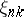,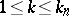,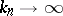,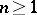, in which(forming the-th series) are mutually independent for any. The simplest sequence of series corresponds to the case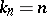. In the limit theorems of probability theory, a fundamental problem is to determine the limiting behaviour, as, of the distributions of the random variables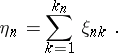(1)

Under suitable conditions the class of limit distributions for such sequences coincides with the class of infinitely-divisible distributions (cf. Infinitely-divisible distribution). Suppose that the sequence of seriessatisfies the condition of uniform asymptotic negligibility, that is,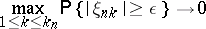(2)

as. Thenis said to form a null sequence of series. The set of distributions that are the limit distributions (in the sense of weak convergence) for the random variables (1), whereis a null sequence of series satisfying the condition of uniform asymptotic negligibility, coincides with the set of infinitely-divisible distributions.

There are conditions for the convergence of the distributions ofto a given infinitely-divisible distribution (see ). In particular, the condition for convergence to a normal distribution has the following form.

Letbe a sequence of series, and let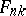be the distribution function of. Forto satisfy (2), and for the distribution of the sums (2) to be weakly convergent to the normal distribution with parametersand, it is necessary and sufficient that for any fixedthe following conditions hold:

1)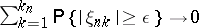,

2)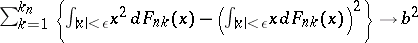,

3)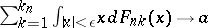.

The study of limit distributions for the normalized partial sums of a sequence of independent random variables is a special case of the study of sequences of series.

As regards sequences of series see also: Infinitely-divisible distribution; Law of large numbers; and Limit theorems. For example, in classical versions of the central limit theorem and the law of large numbers, one considers special cases of sequences of series, formed by random variables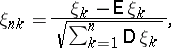where the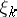are independent random variables.

How to Cite This Entry:
Sequence of series. Encyclopedia of Mathematics. URL: http://encyclopediaofmath.org/index.php?title=Sequence_of_series&oldid=48672
This article was adapted from an original article by N.G. Ushakov (originator), which appeared in Encyclopedia of Mathematics - ISBN 1402006098. See original article